# Aptitude - Geometry

## Point

A point is an exact location

## Line Segment

The straight path between two points A and B is called a line segment AB. A line segment has two end points.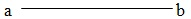## Ray

On extending a line segment AB indefinitely in one direction we get the ray AB. Ray AB has one end point, namely A.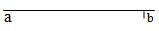## LINE

A line segment AB extended indefinitely in both directions is called line AB.1. A line contains infinitely many points.

2. Through a given points , infinitaly many lines can be drawn.

3. One and only one line can be drawn to pass through two given points A and B.

4. Two line meet in a point.

5. Two planes meet in a line.

### Collinear

In the given figure, the points A,B,C are collinear.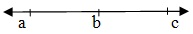### Concurrent Lines

Three or more lines intersecting at the same points are called concurrent lines.

## Angle

Two rays OA and OB having a common end points O form angle AOB, written as ∠AOB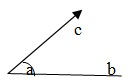### Measure of an Angle

The amount of turning from OA to OB is called the measure of ∠AOB written as m(∠AOB).

### An angle of 360°

If a ray OA starting from its original position OA , rotates about O in anticlockwise direction and after a complete rotation comes back to its original position , then we say that it has rotated through 360. This complete rotation is divided into 360° equal parts. Then, each part is called 1 degree , written as 1°

1° = 60 minutes, written as 60'

1 minute = 60 seconds, written as 60"

### Types of Angle

1. Right angle - An angle whose measure is 90° is called a right angle.

2. Acute angle - An angle whose measure is less than 90° is called an acute angle.

3. Obtuse angle - An angle whose measure is more than 90° but less than 180°, is called an obtues angle.

4. Straight angle - An angle whose measure is 180° is called a Straight angle.

5. Reflex angle - An angle whose measure is more than 180° but less than 360°, is called a Reflex angle.

6. Complete angle - An angle whose measure is 360°, is called a complete angle.

7. Equal angle - Two angles are said to be equal , if they have the same measure.

8. Complementary angleTwo angles are said to be complementary if the sum of their measures is 90. For example, angles measuring 65° and 25° are complementary angle.

9. Supplementary angle - Two angle are said to be supplementary if the sum of their measures is 180°. For example, angles measures 70° and 110° are supplementary.

10. Adjacent angle - Two angles are called adjacent angle if they have the same vertex and a common arm such that non-common arms are on either side of the comman arm. In the given figure , ∠AOC and ∠BOC are adjacent angle.## Important Results

If a ray stands on a line , than the sum of two adjacent angle so formed is 180° In the given figure , ray CP stands on line AB.```∴ ∠ACD + ∠BCD = 180°.
```

The sum of all angle formed on the same side of a line at a given point on the line is 180°. In the given figure four angle are formed on the same side of AOB.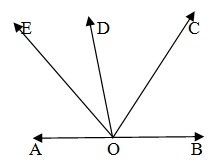```
∴ ∠AOE + ∠EOD + ∠DOC + ∠COD = 180°.
```

The sum of all angle around a point is 360° In the given figure five angle are formed around a point O.```∴∠AOB + ∠BOC + ∠COD + ∠DOE + ∠EOA=360°.
```

## Vertically Opposite Angles

If two lines A Band CD intersect at a point O, then AOC , BOD and BOC , AOD are two pair of vertically opposites angle Vertically opposite angle are always equal.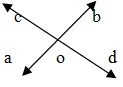```∴ ∠AOC = ∠BOD and ∠AOD = ∠BOC
```

## Parallel Lines

If two lines lie in the same plane and do not intersect when produced on either side then such lines are said to be paralleled and we write , L||m.

## Traversal line cutting parallel linesLet two parallel lines AB and CD be cut by a transversal EF. Then

Corresponding angle are equal

```(∠1 = ∠5), (∠4= ∠8 ), (∠2 = ∠6) , (∠3 = ∠7)
```

Alternate interior angles are equal.

```(∠3 =∠5 )  and  (∠4 =∠6 )
```

Consective interior angles are supplementary

```∠4+∠5 = 180° and ∠3 +∠6 = 180°.
```

## Triangle

A figure bounded by three straight lines is called a triangle. In the given figure , we have ∆ABC; ∆ABC having three vertices A,B,C. In has three angles, namely ∠A,∠B and ∠C. It has three sides , namely AB, AC and BC.## Types of Triangle

1. A triangle having all sides equal is called an equilateral triangle.

2. A triangle having two sides equal, is called an isosceles triangle.

3. A triangle having all sides of different lengths,is called a scalene triangle.

4. A triangle one of whose angles measures 90°,is called a right triangle.

5. A triangle one of whose angle lies between 90° and 180° is called an obtuse triangle.

6. A triangle each of whose angle is acute, is called an acute triangle.

7. The sum of all sides of a triangle is called the perimeter of the triangle.

8. The sum of two sides of a triangle is greater than the third side.

9. In a right angled ABC in which ∠B = 90°, we have AC2 =AB2+BC2. This is called Pythagoras Theorem.

A figure bounded by four straight line is called a quadrilateral. The sum of all angles of a quadrilateral is 360°.

1. Rectangle - A quadrilateral is called a rectangle, if its opposite side are equal and each of its angle is 90°. In given fig. ABCD is a rectangle.2. Square - A quadrilateral is called a square, if all of its sides are equal and each of its angles measures 90°. In given fig. ABCD is square in which AB = BC = CD = DA.3. Parallelogram - A quadrilateral is called a parallelogram, if its opposite sides are parallel. In given fig. ABCD is a parallelogram in which AB = DC & AD = BC.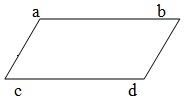4. Rhombus - A parallelogram having all sides equal is called a rhombus. In given fig. ABCD is a rhombus in which AB =BC =CD=DA, AB || DC and AD || BC.## Important Facts

1. A quadrilateral is a rectangle if opposite sides are equal and its diagonals are equal.

2. A quadrilateral is a Square if all sides are equal and the diagonal are equal.

3. A quadrilateral is a parallelogram, if opposite sides are equal.

4. A quadrilateral is a parallelogram but not a rectangle, if opposite sides are equal but the diagonals are not equal.

5. A quadrilateral is a rhombus but not a square if all their sides are equal and the diagonals are not equal.

1. In a parallelogram, we have

1. Opposite sides are equal.

2. Opposite angles are equal.

3. Each diagonal bisects the parallelogram.

4. Diagonals of a parallelogram bisect each other.

2. Diagonals of a rectangle are equal.

3. Diagonals of a rhombus bisect each other at right angles.

## Results on Circle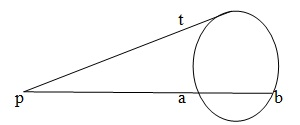1. The perpendicular from the center to a chord bisects the chord.

2. There is one and only one circle passing through three non collinear points.

3. Angle in a semi circle is a right angle.

4. Opposite angles of a cyclic quadrilateral are supplementary.

5. Angle in the same segment of a circle is equal.

6. The tangent at any point of a circle is perpendicular to the radius through the point of contact.

7. Two tangent to a circle from a point outside it are equal.

8. If PT is a tangent to a circle and PAB is a secant, Then PA x PB= PT2

## Solved Examples

Solved Examples
aptitude_geometry.htm